# RS Aggarwal Solutions for Class 8 Maths Chapter 19 - Three-dimensional Figures Exercise 19B

Students can refer and download the RS Aggarwal Solutions for the Exercise 19B of Class 8 Maths Chapter 19, Three-Dimensional Figures from the link available here. BYJU’S expert team has solved the question given in this exercise in a comprehensive manner based on the understanding abilities of students. By practising the RS Aggarwal Solutions for Class 8, students will be able to grasp the concepts correctly. This exercise is based on Euler’s relation to three-dimensional figures. Students who wish to score high in the exams are advised to solve the RS Aggarwal textbook on a daily basis.

## Download PDF of RS Aggarwal Solutions for Class 8 Chapter 19 – Three-Dimensional Figures – Exercise 19B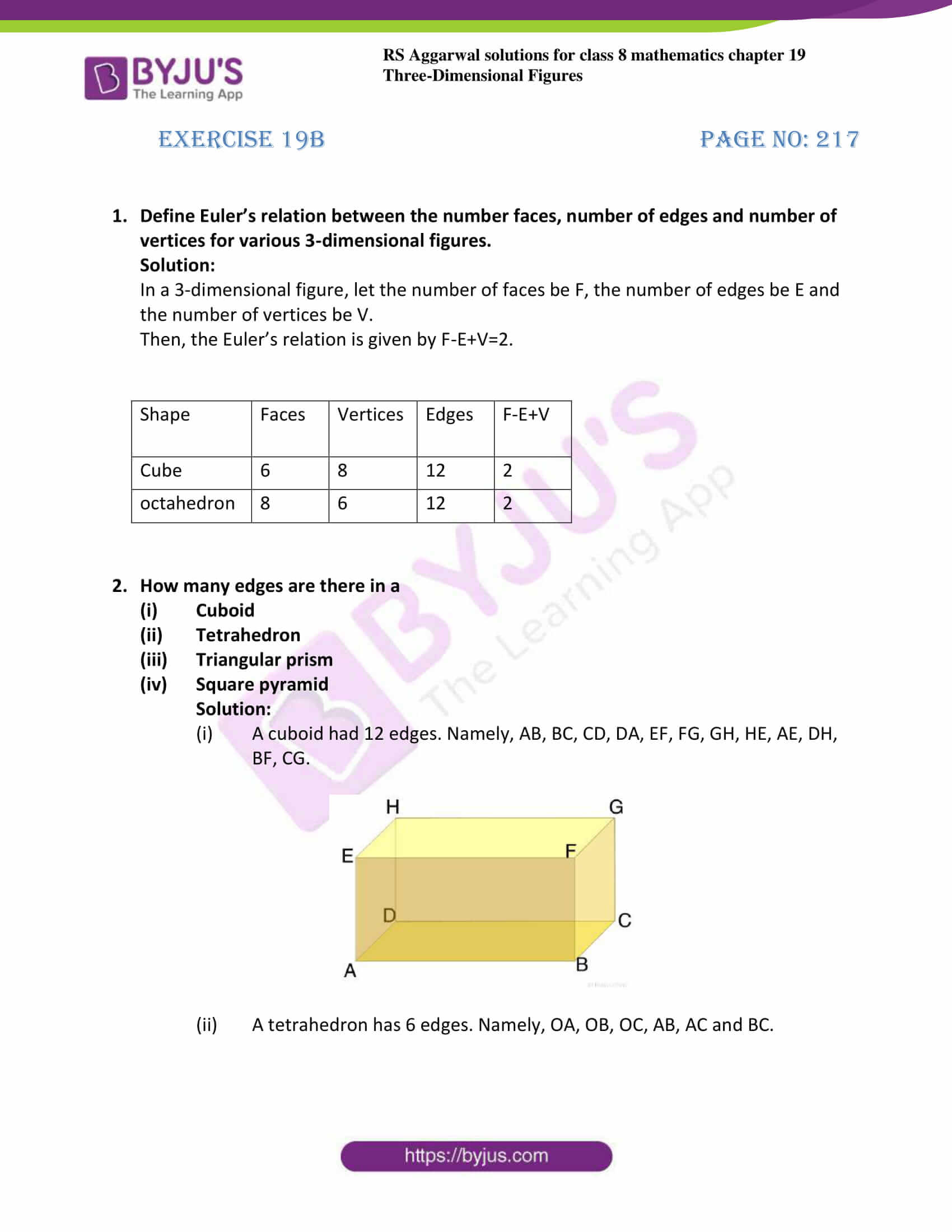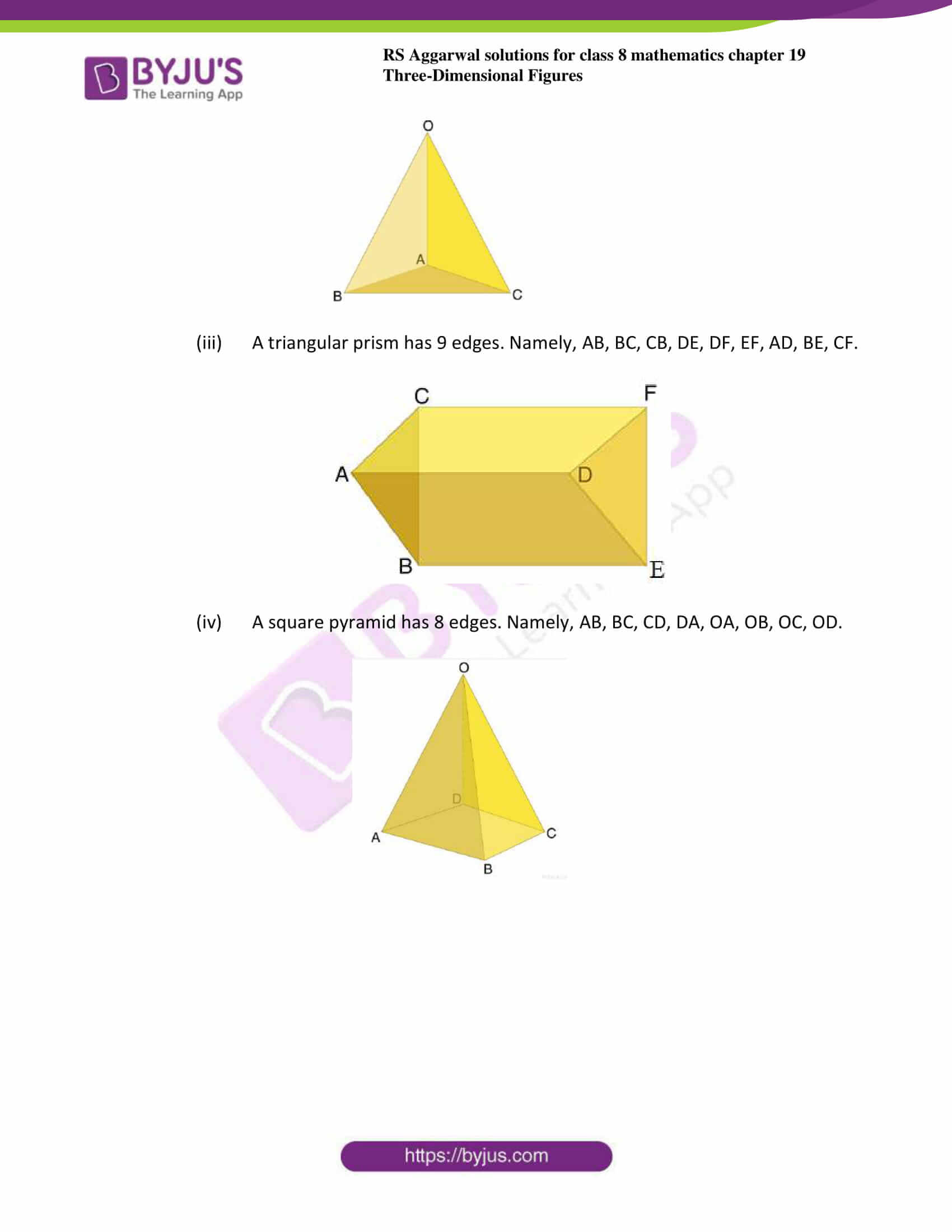### Access answers to Maths RS Aggarwal Solutions for Class 8 Chapter 19 – Three-Dimensional Figures Exercise 19B

1. Define Euler’s relation between the number faces, number of edges and number of vertices for various 3-dimensional figures.

Solution:

In a 3-dimensional figure, let the number of faces be F, the number of edges be E and the number of vertices be V.

Then, the Euler’s relation is given by F-E+V=2.

 Shape Faces Vertices Edges F-E+V Cube 6 8 12 2 octahedron 8 6 12 2

2. How many edges are there in a

1. Cuboid
2. Tetrahedron
3. Triangular prism
4. Square pyramid

Solution:

1. A cuboid had 12 edges. Namely, AB, BC, CD, DA, EF, FG, GH, HE, AE, DH, BF, CG.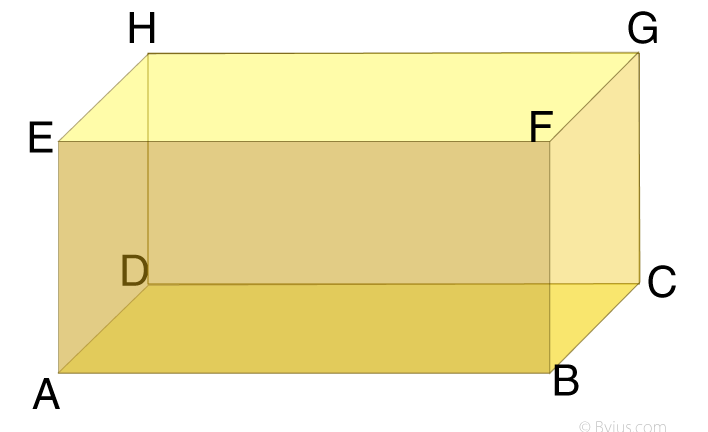2. A tetrahedron has 6 edges. Namely, OA, OB, OC, AB, AC and BC.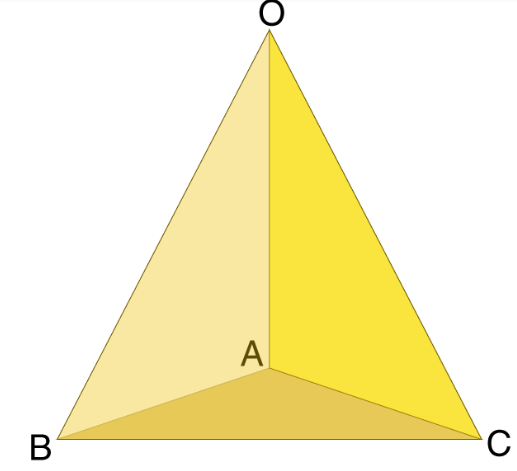3. A triangular prism has 9 edges. Namely, AB, BC, CB, DE, DF, EF, AD, BE, CF.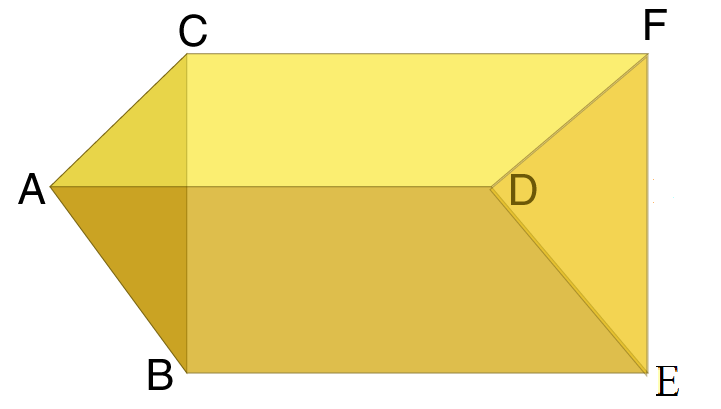4. A square pyramid has 8 edges. Namely, AB, BC, CD, DA, OA, OB, OC, OD.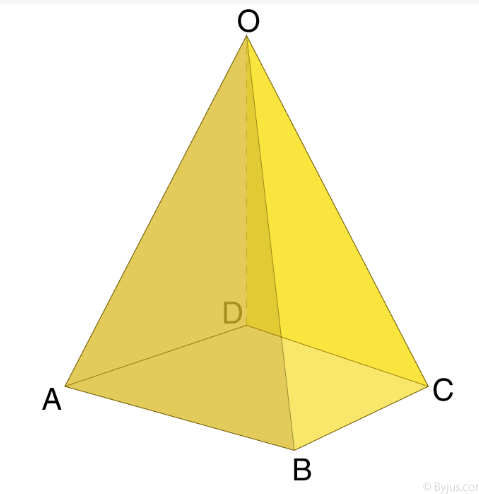### Access another exercise of RS Aggarwal Solutions for Class 8 Maths Chapter 19 – Three-Dimensional Figures

Exercise 19A Solutions 4 questions

## RS Aggarwal Solutions for Class 8 Maths Chapter 19 – Three-Dimensional Figures Exercise 19B

Exercise 19B of RS Aggarwal Solutions for Chapter 19, Three-Dimensional figures deals with the basic concepts related to 3-dimensional figures. We can say that this exercise mainly deals with the Euler’s relation to three-dimensional figures that the students have learnt in this chapter.

The RS Aggarwal Solutions help students to learn the topics effectively as it provides solutions to all the questions present in the RS Aggarwal textbook. Those who aim to score high marks in the annual are recommended to practice all the questions present in RS Aggarwal as many times as possible.